GeeksforGeeks App
Open AppBrowser
Continue

# How to Generate mesh in Python with Gmsh module?

In this article, we will cover how to Generate meshes using Gmsh module in Python.

## What is Mesh?

A connected 2D, 3D, or multi-dimension structure that is made up of points, lines, and curves is called mesh. In real-world meshes are made up of many different materials such as metals, fibers, and ductile materials. For example, the image is shown below:

3D mesh: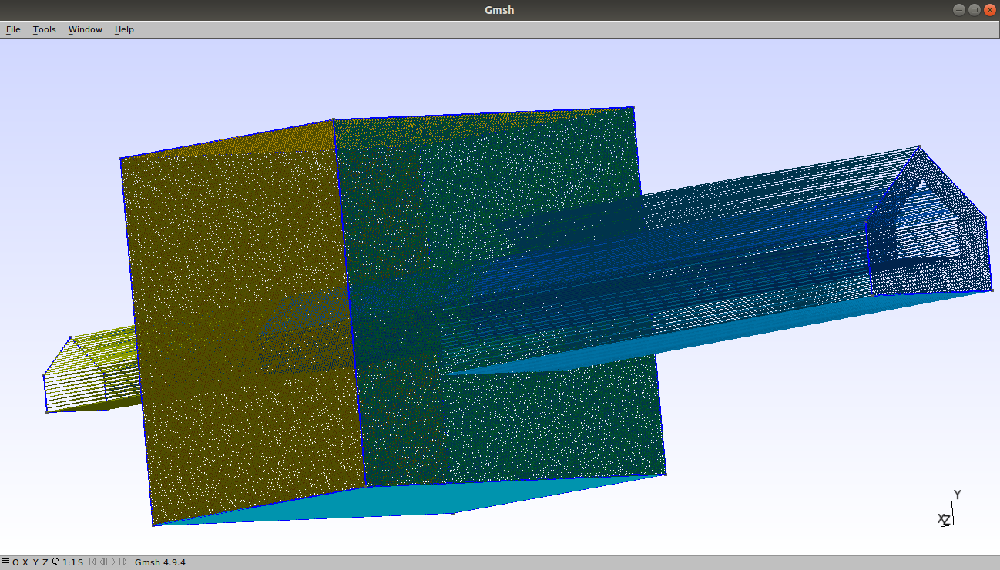### Methods:

After understanding mesh and functions it’s time to create the above mesh. So without further delay, let’s jump right in.

## Module required:

Gmsh library: It is a script of promotion and API Python wrapper for gmsh.

`pip install gmsh`

Sys library: It is a script of promotion and API Python wrapper for sys.

`pip install sys`

## Stepwise implementation

Step1: Import modules, initialize gmsh.

## Python3

 `# Import modules:``import` `gmsh``import` `sys` `# Initialize gmsh:``gmsh.initialize()`

Step2: The above mesh is made up of three shapes a cube and two pentagons. So first we create a cube then both pentagons. To create a cube we need to create 8 points, 12 edges/lines, 6 faces, and their surfaces. To create points use the code given below.

## Python3

 `# Import modules:``import` `gmsh``import` `sys` `# Initialize gmsh:``gmsh.initialize()` `# cube points:``lc ``=` `1e``-``2``point1 ``=` `gmsh.model.geo.add_point(``0``, ``0``, ``0``, lc)``point2 ``=` `gmsh.model.geo.add_point(``1``, ``0``, ``0``, lc)``point3 ``=` `gmsh.model.geo.add_point(``1``, ``1``, ``0``, lc)``point4 ``=` `gmsh.model.geo.add_point(``0``, ``1``, ``0``, lc)``point5 ``=` `gmsh.model.geo.add_point(``0``, ``1``, ``1``, lc)``point6 ``=` `gmsh.model.geo.add_point(``0``, ``0``, ``1``, lc)``point7 ``=` `gmsh.model.geo.add_point(``1``, ``0``, ``1``, lc)``point8 ``=` `gmsh.model.geo.add_point(``1``, ``1``, ``1``, lc)` `# Create the relevant Gmsh data structures``# from Gmsh model.``gmsh.model.geo.synchronize()` `# Generate mesh:``gmsh.model.mesh.generate()` `# Write mesh data:``gmsh.write(``"GFG.msh"``)` `# Creates  graphical user interface``if` `'close'` `not` `in` `sys.argv:``    ``gmsh.fltk.run()` `# It finalize the Gmsh API``gmsh.finalize()`

Output: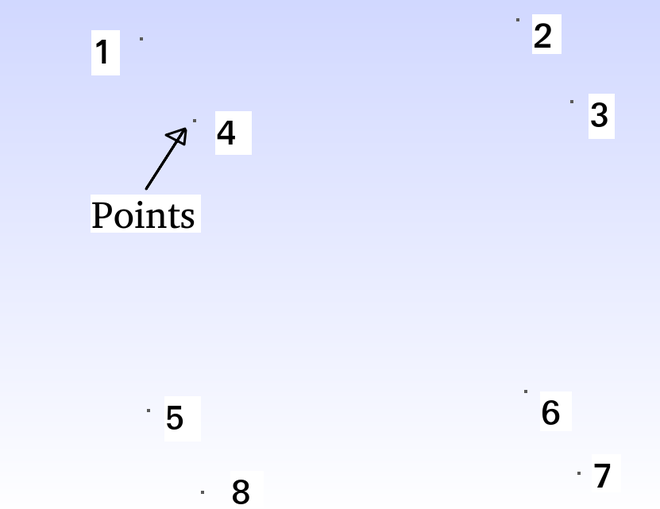Step3: After creating points, we are able to create lines from these points using the add_line() method. To create lines use the code given below.

## Python3

 `# Import modules:``import` `gmsh``import` `sys` `# Initialize gmsh:``gmsh.initialize()` `# cube points:``lc ``=` `1e``-``2``point1 ``=` `gmsh.model.geo.add_point(``0``, ``0``, ``0``, lc)``point2 ``=` `gmsh.model.geo.add_point(``1``, ``0``, ``0``, lc)``point3 ``=` `gmsh.model.geo.add_point(``1``, ``1``, ``0``, lc)``point4 ``=` `gmsh.model.geo.add_point(``0``, ``1``, ``0``, lc)``point5 ``=` `gmsh.model.geo.add_point(``0``, ``1``, ``1``, lc)``point6 ``=` `gmsh.model.geo.add_point(``0``, ``0``, ``1``, lc)``point7 ``=` `gmsh.model.geo.add_point(``1``, ``0``, ``1``, lc)``point8 ``=` `gmsh.model.geo.add_point(``1``, ``1``, ``1``, lc)` `# Edge of cube:``line1 ``=` `gmsh.model.geo.add_line(point1, point2)``line2 ``=` `gmsh.model.geo.add_line(point2, point3)``line3 ``=` `gmsh.model.geo.add_line(point3, point4)``line4 ``=` `gmsh.model.geo.add_line(point4, point1)``line5 ``=` `gmsh.model.geo.add_line(point5, point6)``line6 ``=` `gmsh.model.geo.add_line(point6, point7)``line7 ``=` `gmsh.model.geo.add_line(point7, point8)``line8 ``=` `gmsh.model.geo.add_line(point8, point5)``line9 ``=` `gmsh.model.geo.add_line(point4, point5)``line10 ``=` `gmsh.model.geo.add_line(point6, point1)``line11 ``=` `gmsh.model.geo.add_line(point7, point2)``line12 ``=` `gmsh.model.geo.add_line(point3, point8)` `# Create the relevant Gmsh data structures``# from Gmsh model.``gmsh.model.geo.synchronize()` `# Generate mesh:``gmsh.model.mesh.generate()` `# Write mesh data:``gmsh.write(``"GFG.msh"``)` `# Creates  graphical user interface``if` `'close'` `not` `in` `sys.argv:``    ``gmsh.fltk.run()` `# It finalize the Gmsh API``gmsh.finalize()`

Output: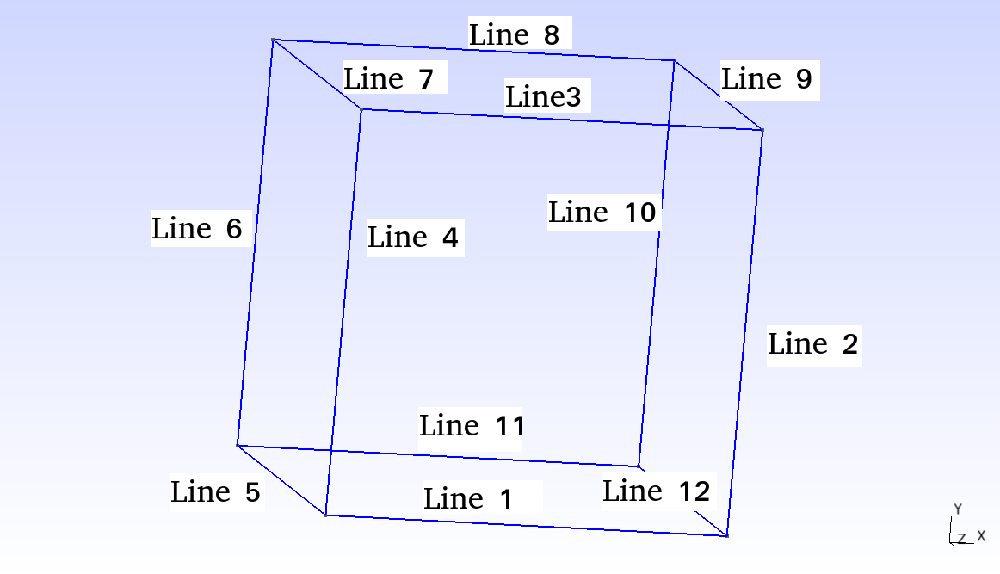Step 4: Next we create faces and surfaces but before that one should understand two methods more clearly that are add_curve_loop() and add_plane_surface().  In the above output, it looks like faces are generated but it is not true. So to create solid faces we use the add_curve_loop() then we use the add_plane_surface() method so that we get a solid face with different colors.

• add_curve_loop([line1, line2, line3, line4]):  It required a list of lines in close loop format. For example: [line1(point1 to point2) -> line2(point2 to point3) -> line3(point3 to point4) -> line4(point4 to point1)], In this way point1 is connected to point4.  So a face of the above square is produced. If we are moving in opposite directions of line i.e line1 = (point1 to point2) and if we are moving from (point2 to point1) then line1 is written as “-line1” in  add_curve_loop() function because of the opposite direction.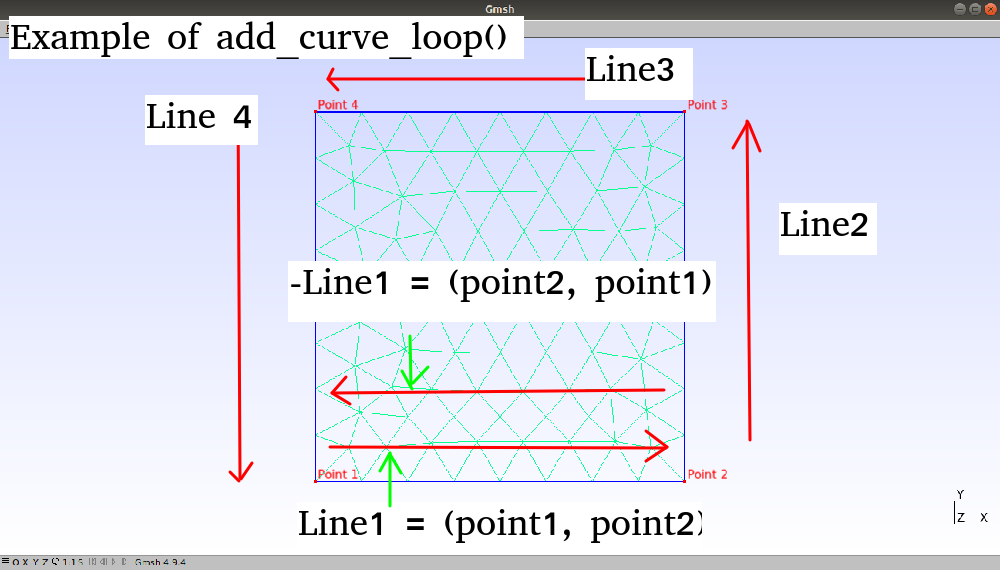• add_plane_surface(): If only one face is passed as a parameter then the function creates an only surface on the face. If two faces are passed as parameters in the list then the function connects faces if it is possible i.e if the faces lie in the same plane.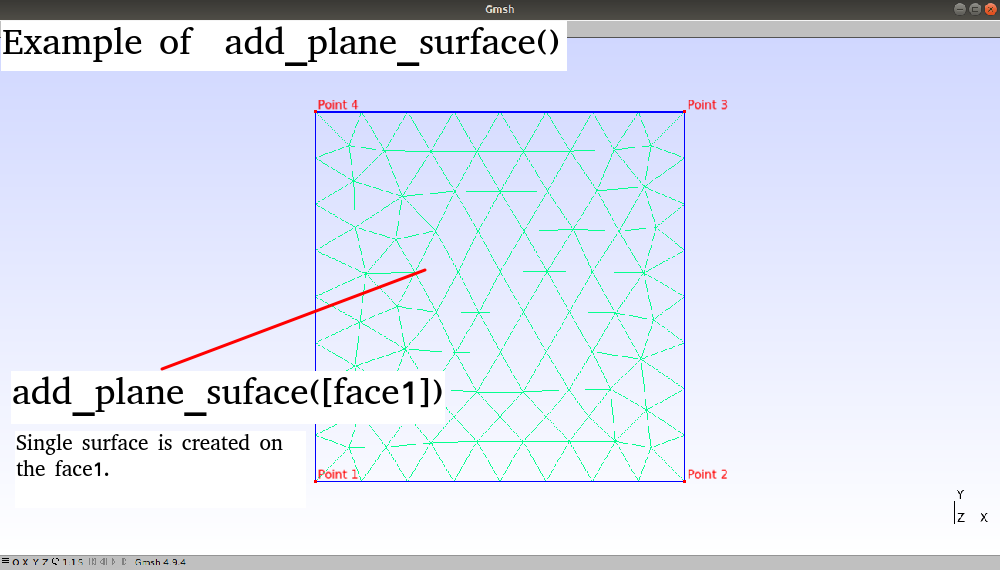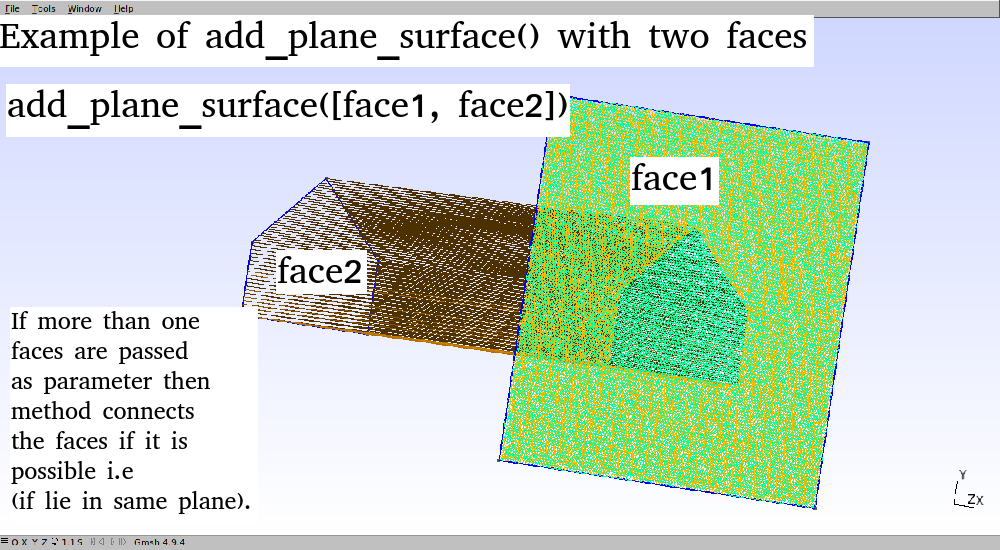After understanding both methods cube can be created using the given code below.

## Python3

 `# Import modules:``import` `gmsh``import` `sys` `# Initialize gmsh:``gmsh.initialize()` `# cube points:``lc ``=` `1e``-``2``point1 ``=` `gmsh.model.geo.add_point(``0``, ``0``, ``0``, lc)``point2 ``=` `gmsh.model.geo.add_point(``1``, ``0``, ``0``, lc)``point3 ``=` `gmsh.model.geo.add_point(``1``, ``1``, ``0``, lc)``point4 ``=` `gmsh.model.geo.add_point(``0``, ``1``, ``0``, lc)``point5 ``=` `gmsh.model.geo.add_point(``0``, ``1``, ``1``, lc)``point6 ``=` `gmsh.model.geo.add_point(``0``, ``0``, ``1``, lc)``point7 ``=` `gmsh.model.geo.add_point(``1``, ``0``, ``1``, lc)``point8 ``=` `gmsh.model.geo.add_point(``1``, ``1``, ``1``, lc)` `# Edge of cube:``line1 ``=` `gmsh.model.geo.add_line(point1, point2)``line2 ``=` `gmsh.model.geo.add_line(point2, point3)``line3 ``=` `gmsh.model.geo.add_line(point3, point4)``line4 ``=` `gmsh.model.geo.add_line(point4, point1)``line5 ``=` `gmsh.model.geo.add_line(point5, point6)``line6 ``=` `gmsh.model.geo.add_line(point6, point7)``line7 ``=` `gmsh.model.geo.add_line(point7, point8)``line8 ``=` `gmsh.model.geo.add_line(point8, point5)``line9 ``=` `gmsh.model.geo.add_line(point4, point5)``line10 ``=` `gmsh.model.geo.add_line(point6, point1)``line11 ``=` `gmsh.model.geo.add_line(point7, point2)``line12 ``=` `gmsh.model.geo.add_line(point3, point8)` `# faces of cube:``face1 ``=` `gmsh.model.geo.add_curve_loop([line1, line2, line3, line4])``face2 ``=` `gmsh.model.geo.add_curve_loop([line5, line6, line7, line8])``face3 ``=` `gmsh.model.geo.add_curve_loop([line9, line5, line10, ``-``line4])``face4 ``=` `gmsh.model.geo.add_curve_loop([line9, ``-``line8, ``-``line12, line3])``face5 ``=` `gmsh.model.geo.add_curve_loop([line6, line11, ``-``line1, ``-``line10])``face6 ``=` `gmsh.model.geo.add_curve_loop([line11, line2, line12, ``-``line7])` `# surfaces of cube:``gmsh.model.geo.add_plane_surface([face1])``gmsh.model.geo.add_plane_surface([face2])``gmsh.model.geo.add_plane_surface([face3])``gmsh.model.geo.add_plane_surface([face4])``gmsh.model.geo.add_plane_surface([face5])``gmsh.model.geo.add_plane_surface([face6])` `# Create the relevant Gmsh data structures``# from Gmsh model.``gmsh.model.geo.synchronize()` `# Generate mesh:``gmsh.model.mesh.generate()` `# Write mesh data:``gmsh.write(``"GFG.msh"``)` `# Creates  graphical user interface``if` `'close'` `not` `in` `sys.argv:``    ``gmsh.fltk.run()` `# It finalize the Gmsh API``gmsh.finalize()`

Output: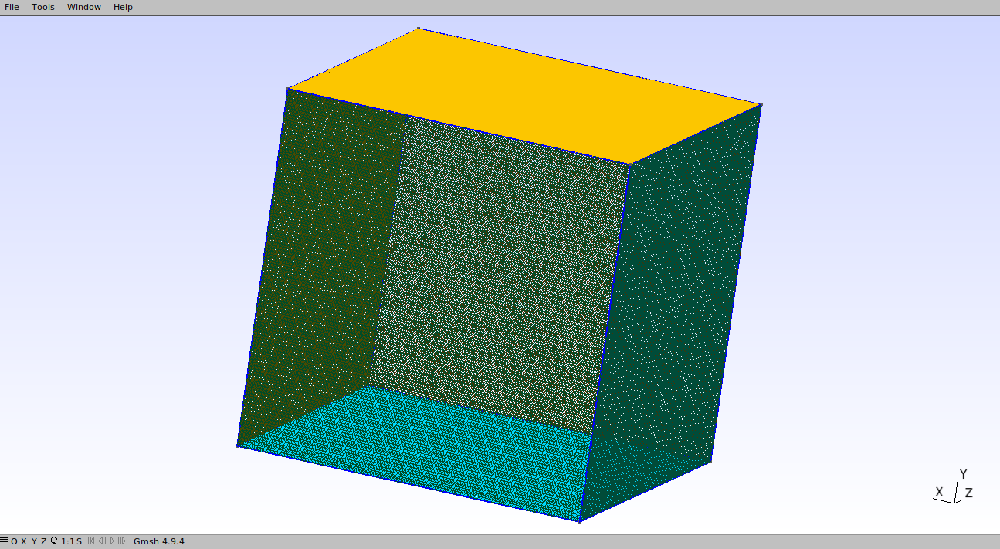Step5: Now the final step is to create both pentagons and connect them. To do so one should need to create their points and lines using proper measurement it is better to draw figures on paper first then code points according. Here we create two pentagons one bigger and one smaller using add_point(), add_line() functions.  After that, we will create surfaces of both the pentagons using add_curve_loop() and then connect the bigger and smaller pentagon face with the cube face using add_plane_surface.  At last, we will connect both pentagon’s faces with each other using the add_plane_surface() function.

## Python3

 `# Import modules:``import` `gmsh``import` `sys` `# Initialize gmsh:``gmsh.initialize()` `# cube points:``lc ``=` `1e``-``2``point1 ``=` `gmsh.model.geo.add_point(``0``, ``0``, ``0``, lc)``point2 ``=` `gmsh.model.geo.add_point(``1``, ``0``, ``0``, lc)``point3 ``=` `gmsh.model.geo.add_point(``1``, ``1``, ``0``, lc)``point4 ``=` `gmsh.model.geo.add_point(``0``, ``1``, ``0``, lc)``point5 ``=` `gmsh.model.geo.add_point(``0``, ``1``, ``1``, lc)``point6 ``=` `gmsh.model.geo.add_point(``0``, ``0``, ``1``, lc)``point7 ``=` `gmsh.model.geo.add_point(``1``, ``0``, ``1``, lc)``point8 ``=` `gmsh.model.geo.add_point(``1``, ``1``, ``1``, lc)` `# Edge of cube:``line1 ``=` `gmsh.model.geo.add_line(point1, point2)``line2 ``=` `gmsh.model.geo.add_line(point2, point3)``line3 ``=` `gmsh.model.geo.add_line(point3, point4)``line4 ``=` `gmsh.model.geo.add_line(point4, point1)``line5 ``=` `gmsh.model.geo.add_line(point5, point6)``line6 ``=` `gmsh.model.geo.add_line(point6, point7)``line7 ``=` `gmsh.model.geo.add_line(point7, point8)``line8 ``=` `gmsh.model.geo.add_line(point8, point5)``line9 ``=` `gmsh.model.geo.add_line(point4, point5)``line10 ``=` `gmsh.model.geo.add_line(point6, point1)``line11 ``=` `gmsh.model.geo.add_line(point7, point2)``line12 ``=` `gmsh.model.geo.add_line(point3, point8)` `# faces of cube:``face1 ``=` `gmsh.model.geo.add_curve_loop([line1, line2, line3, line4])``face2 ``=` `gmsh.model.geo.add_curve_loop([line5, line6, line7, line8])``face3 ``=` `gmsh.model.geo.add_curve_loop([line9, line5, line10, ``-``line4])``face4 ``=` `gmsh.model.geo.add_curve_loop([line9, ``-``line8, ``-``line12, line3])``face5 ``=` `gmsh.model.geo.add_curve_loop([line6, line11, ``-``line1, ``-``line10])``face6 ``=` `gmsh.model.geo.add_curve_loop([line11, line2, line12, ``-``line7])` `# surfaces of cube:``gmsh.model.geo.add_plane_surface([face1])``gmsh.model.geo.add_plane_surface([face2])``gmsh.model.geo.add_plane_surface([face3])``gmsh.model.geo.add_plane_surface([face4])``gmsh.model.geo.add_plane_surface([face5])``gmsh.model.geo.add_plane_surface([face6])` `# Points of bigger petagon:``point9 ``=` `gmsh.model.geo.add_point(``0.3``, ``0.3``, ``-``2``, lc)``point10 ``=` `gmsh.model.geo.add_point(``0.7``, ``0.3``, ``-``2``, lc)``point11 ``=` `gmsh.model.geo.add_point(``0.7``, ``0.5``, ``-``2``, lc)``point12 ``=` `gmsh.model.geo.add_point(``0.5``, ``0.7``, ``-``2``, lc)``point13 ``=` `gmsh.model.geo.add_point(``0.3``, ``0.5``, ``-``2``, lc)` `# Points of smaller petagon:``point14 ``=` `gmsh.model.geo.add_point(``0.4``, ``0.4``, ``2``, lc)``point15 ``=` `gmsh.model.geo.add_point(``0.6``, ``0.4``, ``2``, lc)``point16 ``=` `gmsh.model.geo.add_point(``0.6``, ``0.5``, ``2``, lc)``point17 ``=` `gmsh.model.geo.add_point(``0.5``, ``0.6``, ``2``, lc)``point18 ``=` `gmsh.model.geo.add_point(``0.4``, ``0.5``, ``2``, lc)` `# lines of bigger pentagon:``line13 ``=` `gmsh.model.geo.add_line(point9, point10)``line14 ``=` `gmsh.model.geo.add_line(point10, point11)``line15 ``=` `gmsh.model.geo.add_line(point11, point12)``line16 ``=` `gmsh.model.geo.add_line(point12, point13)``line17 ``=` `gmsh.model.geo.add_line(point13, point9)` `# lines of smaller pentagon:``line18 ``=` `gmsh.model.geo.add_line(point14, point15)``line19 ``=` `gmsh.model.geo.add_line(point15, point16)``line20 ``=` `gmsh.model.geo.add_line(point16, point17)``line21 ``=` `gmsh.model.geo.add_line(point17, point18)``line22 ``=` `gmsh.model.geo.add_line(point18, point14)` `# face of bigger pentagon.``face7 ``=` `gmsh.model.geo.add_curve_loop([line13, line14, line15, line16, line17])` `# face of smaller pentagon.``face8 ``=` `gmsh.model.geo.add_curve_loop([line18, line19, line20, line21, line22])` `# connection of cube faces with pentagon``# and bigger pentagon with smaller.``gmsh.model.geo.add_plane_surface([face1, face7])``gmsh.model.geo.add_plane_surface([face2, face8])``gmsh.model.geo.add_plane_surface([face7, face8])` `# Create the relevant Gmsh data structures``# from Gmsh model.``gmsh.model.geo.synchronize()` `# Generate mesh:``gmsh.model.mesh.generate()` `# Write mesh data:``gmsh.write(``"GFG.msh"``)` `# Creates  graphical user interface``if` `'close'` `not` `in` `sys.argv:``    ``gmsh.fltk.run()` `# It finalize the Gmsh API``gmsh.finalize()`

Output: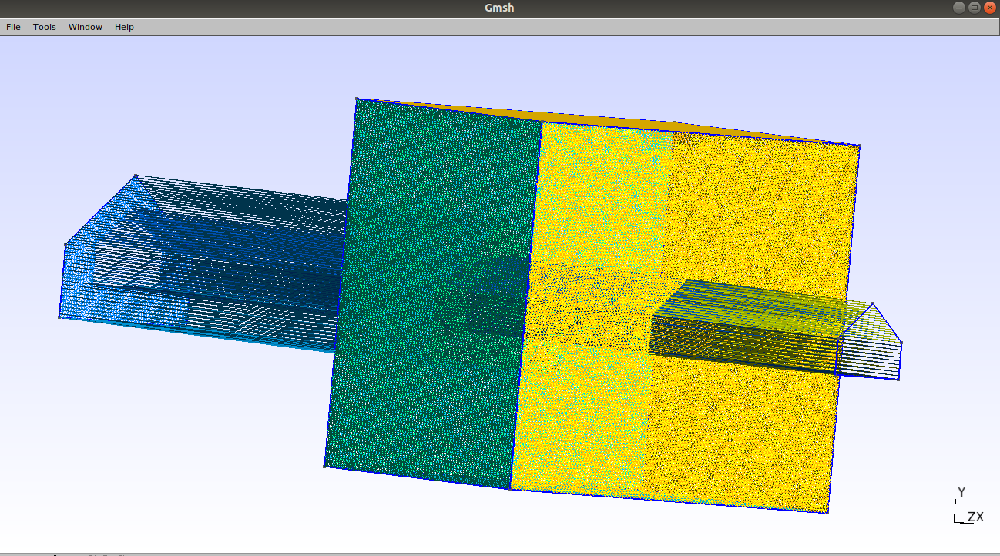My Personal Notes arrow_drop_up